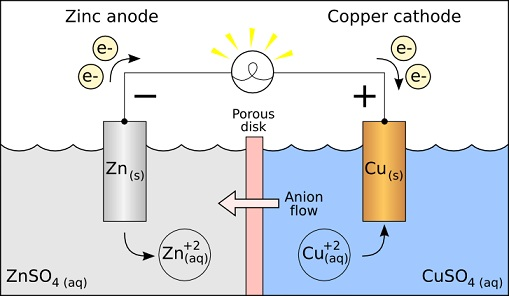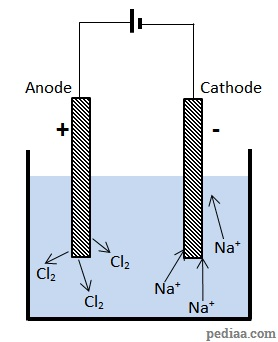# Difference Between Anode and Cathode

## Main Difference – Anode vs. Cathode

The terms cathode and anode are used to refer to terminals of a polarised electrical device. The main difference between anode and cathode is that, in general, anode is the terminal where the (conventional) current flows into a device from outside, whereas cathode is the terminal where (conventional) current flows out of the device. However, the usage is not strictly followed in some instances, because when a device is able to undergo a reversible process, the same terminal that was called the “anode” could be now called the “cathode”. Undoubtedly, this may lead to confusion and it is advisable to adapt to the general usage in the specific field. In this article, we will look at several scenarios where these terms are used, and explore their usage in terms of the processes that occur in these devices.

## What is an Anode

Anode is the terminal where the (conventional) current flows into a device from outside. This means that electrons flow out of the device at the anode.

## What is a Cathode

Cathode is the terminal where the (conventional) current flows out of a device. This means that electrons flow into this terminal from the outside.

## Galvanic/Voltaic Cells

The setup of a galvanic cell is shown below:A Galvanic cell

In a Galvanic cell, one of the electrodes is at a higher reduction potential than the other. The electrode with a higher reduction potential has a stronger ability to gain electrons, so electrons flow into it from the other electrode. In the cell drawn above, copper has a higher reduction potential than zinc, so it draws electrons from the zinc electrode. This accompanies two reactions. At the zinc electrode, the zinc dissociates into Zn2+ ions and electrons. In other words, zinc is being oxidised (it loses electrons).$\mathrm{Zn\rightarrow Zn^{2+}+2e^-}$

The electrons lost by zinc flow across the wires onto the copper electrode. Here, the incoming electrons combine with Cu2+ ions and form copper atoms. Copper is being reduced (it gains electrons):$\mathrm{Cu^{2+}+2e^-\rightarrow Cu}$

Here, electrons flow “out of the device” from the zinc terminal, so the conventional current is flowing into the device here. This makes the zinc terminal the anode. Conventional current flows out of the device at the copper terminal, so that makes copper the cathode. Whenever a device works using redox reactions, the terminal where oxidation happens is the anode, and the electrode where reduction happens is the cathode. This agrees with the description above: zinc (the anode) becomes oxidised and copper (the cathode) gets reduced.

## Electrolytic Cells

In electrolytic cells, a power supply is used to create a current in a liquid containing ions. For instance, we will look at what happens when two electrodes are put into a sample of molten sodium chloride (NaCl, or common salt).Electrolysis of molten sodium chloride

The electrode connected to the positive terminal of the battery attracts the$Cl^-$ anions. Here, these ions give off their electrons, forming chlorine gas.$\mathrm{2Cl^-\rightarrow Cl_2+2e}$

At the electrode connected to the negative terminal, the positive sodium ions gain electrons, forming sodium atoms:$\mathrm{Na^++e\rightarrow Na}$

Here, the terminal that draws current into the device is the electrode connected to the positive terminal of the battery.  Therefore, this is the anode.$Cl^-$ ions lose their electrons here, so this is consistent with the idea that oxidation happens at the anode. Sodium forms at the other electrode where$Na^+$ ions are reduced. Current flows out of the device from this terminal. Therefore, this terminal forms the cathode.

The above two examples should clarify that the terms anode and cathode do not refer to a specific potential, but rather how current flows in the setup. For example, the “positive” electrode in the Galvanic cell is its “cathode”, but the “positive” electrode in the case of electrolysis is its “anode”.

## Difference Between Anode and Cathode

The names “anode” and “cathode” can be given to a terminal depending on whether current flows into that terminal from outside, or whether current flows out of the terminal to the outside. However, because the way currents flow in different situations could be radically different, translating the use of these terms from one situation to another could be confusing. Therefore, it may be necessary to first examine the situation in order to use the terminology properly. If possible, alternative, less ambiguous terms should be used (depending on the situation). We have discussed two particular examples from electrochemistry but the terms “anode” and “cathode” are used in many other fields as well. A few more examples are mentioned in the summary section below.

### Current Flow Direction:

Generally, current flows into the anode from the outside.

Cathode gives current out of the device. This means that outside the device, electrons flow from anode to cathode.

### Redox Reaction:

In devices that rely on redox reactions, oxidation takes place at the anodes.

Whereas reduction takes place at cathodes.

### In Galvanic Cells and Electrolytic Cells:

In Galvanic cells and electrolytic cells, the cathode attracts cations and oxidises them.

The anode attracts anions and reduces them.

### In Electrolysis:

The anode forms the positive terminal in electrolysis

While, the cathode forms the negative terminal in the Galvanic cell.

### In Electron Guns and X-ray Tubes:

In electron guns and X-ray tubes, the part that emits electrons into the device forms the cathode.

Inside the device, the anode collects the electrons.

When normal diodes are connected in forward bias, the anode is the p-side, which is the side connected to the positive side of the battery (it draws current from the cell). Similarly, the cathode forms the n-side.

Although the names of the terminals should be reversed when current flows in reverse bias in a Zener diode, the p-side is still referred to as the “anode” even though technically it gives current to the outside. This is a notable exception, and highlights why the terms “anode” and “cathode” should be avoided, when possible (in this case, it is better to refer to the sides as the p-side and the n-side).

Another source of confusion occurs when battery manufacturers label the negative terminal of a rechargeable battery as the “anode“. When the battery is discharging, the terminology works. However, when the battery is being charged, technically, the terminology should be reversed, as well.

References:

Denker, J. (2004). How to Define Anode and Cathode. Retrieved October 1, 2015, from Welcome to Av8n.com

Image Courtesy:

“Galvanic cell diagram” by Ohio standard (Transferred from en.wikipedia; transferred to Commons by User:Burpelson AFB using CommonsHelper) [CC BY-SA 3.0], via Wikimedia Commons